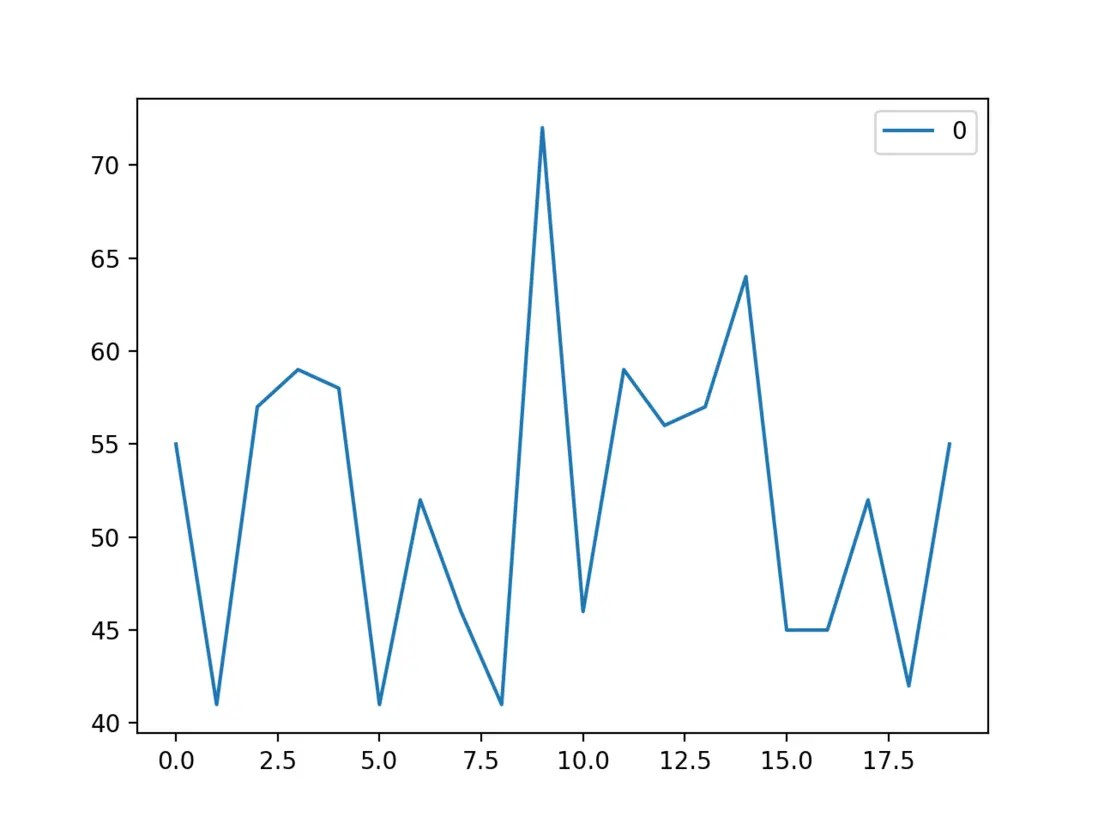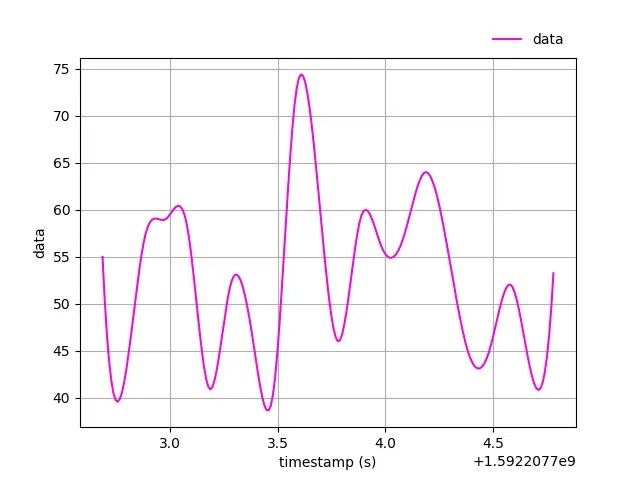# Scientific computing: Python analyzes data to find problems and graphs

Posted Jun 16, 20204 min read

How to analyze or graph the recorded data in Python?

This article will introduce numpy, matplotlib, pandas, and scipy packages for data analysis and graphing.

## Prepare the environment

Anaconda is a Python distribution for scientific computing. It already contains many popular Python packages for scientific computing and data analysis.

You can list existing packages with `conda list`, you will find that there are several packages to be introduced in this article:

``````\$conda list | grep numpy
numpy 1.17.2 py37h99e6662_0

\$conda list | grep "matplot\|seaborn\|plotly"
matplotlib 3.1.1 py37h54f8f79_0
seaborn 0.9.0 py37_0

\$conda list | grep "pandas\|scipy"
pandas 0.25.1 py37h0a44026_0
scipy 1.3.1 py37h1410ff5_0``````

If you already have a Python environment, then pip install them:

``````pip install numpy matplotlib pandas scipy
# pypi mirror:https://mirrors.tuna.tsinghua.edu.cn/help/pypi/``````

## Prepare data

This article assumes data `data0.txt` in the following format:

``````id, data, timestamp
0, 55, 1592207702.688805
1, 41, 1592207702.783134
2, 57, 1592207702.883619
3, 59, 1592207702.980597
4, 58, 1592207703.08313
5, 41, 1592207703.183011
6, 52, 1592207703.281802
...``````

CSV format:comma separated, easy to read and write, Excel can be opened.

After that, we will achieve the following goals together:

• CSV data, numpy reading and calculation
• data column data, matplotlib graphical
• Data column data, scipy interpolation, forming a curve
• Timestamp column data, difference before and after pandas analysis, number per second

numpy can read CSV data directly with `loadtxt`,

``````import numpy as np

# id,(data), timestamp
datas = np.loadtxt(p, dtype=np.int32, delimiter=",", skiprows=1, usecols=(1))``````
• `dtype=np.int32`:data type `np.int32`
• `delimiter=","`:separator ","
• `skiprows=1`:skip the first row
• `usecols=(1)`:read the first column

``````# id,(data, timestamp)
dtype = {'names':('data','timestamp'),'formats':('i4','f8')}
datas = np.loadtxt(path, dtype=dtype, delimiter=",", skiprows=1, usecols=(1, 2))``````

The description of `dtype` is visible: https://numpy.org/devdocs/ref...

## numpy analysis data

numpy calculates the mean and sample standard deviation:

``````# average
data_avg = np.mean(datas)
# data_avg = np.average(datas)

# standard deviation
# data_std = np.std(datas)
# sample standard deviation
data_std = np.std(datas, ddof=1)

print(" avg:{:.2f}, std:{:.2f}, sum:{}".format(
data_avg, data_std, np.sum(datas)))``````

## matplotlib graphical

Just four lines, it can be displayed graphically:

``````import sys

import matplotlib.pyplot as plt
import numpy as np

def _plot(path):
# id,(data), timestamp
datas = np.loadtxt(path, dtype=np.int32, delimiter=",", skiprows=1, usecols=(1))

fig, ax = plt.subplots()
ax.plot(range(len(datas)), datas, label=str(i))
ax.legend()
plt.show()

if __name__ == "__main__":
if len(sys.argv) <2:
sys.exit("python data_plot.py *.txt")
_plot(sys.argv)``````

`ax.plot(x, y, ...)` abscissa `x` the data subscript `range(len(datas))`.

For the complete code, see `data_plot.py` at the Gist address at the end of the article. The running effect is as follows:

``````\$python data_plot.py data0.txt
Args
nonzero:False
size:20
avg:52.15, std:8.57, sum:1043``````Multiple files can be read and displayed together:

``````\$python data_plot.py data*.txt
Args
nonzero:False
size:20
avg:52.15, std:8.57, sum:1043
size:20
avg:53.35, std:6.78, sum:1067``````## scipy interpolate data

`x`, `y` two sets of data, interpolated with scipy, smoothed into a curve:

``````from scipy import interpolate

xnew = np.arange(xvalues, xvalues[-1], 0.01)
ynew = interpolate.interp1d(xvalues, yvalues, kind='cubic')``````

For the complete code, see `data_interp.py` at the Gist address at the end of the article. The running effect is as follows:

``python data_interp.py data0.txt```matplotlib` how to configure, delay, save when visualizing, code and comments are visible.

## pandas analysis data

Here you need to read the timestamp column data,

``````# id, data,(timestamp)
stamps = np.loadtxt(path, dtype=np.float64, delimiter=",", skiprows=1, usecols=(2))``````

numpy calculates the difference between before and after,

``stamps_diff = np.diff(stamps)``

pandas counts the number per second,

``````stamps_int = np.array(stamps, dtype='int')
stamps_int = stamps_int-stamps_int
import pandas as pd
stamps_s = pd.Series(data=stamps_int)
stamps_s = stamps_s.value_counts(sort=False)``````

Method:change the timestamp directly to the number of seconds, and then pandas counts the same value.

For the complete code, see `stamp_diff.py` at the Gist address at the end of the article. The running effect is as follows:

``python stamp_diff.py data0.txt```matplotlib` How to display multiple charts when graphical, also see the code.

## Conclusion